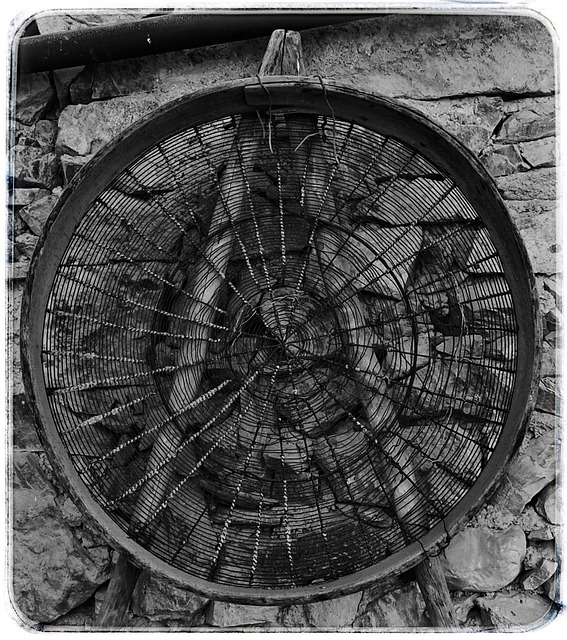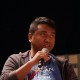# The Sieve of Eratosthenes (Implemented in C)

If you like programming puzzles and challenges you’ll notice that many of them involve prime numbers in one way or another. As such it becomes handy to have a large table of prime numbers ready to go.One of the easiest yet efficient methods to generate a list of prime numbers if the Sieve of Eratosthenes (link to Wikipedia).

Here’s the basic idea:

1. Create a list with all positive integers (starting from 2 as 1 is not considered prime).
2. Start at the first valid number (at this point all are valid) and eliminate all its multiples from the list. So start with 2, and eliminate 4, 6, 8, 10, 12 and so on.
3. Once all the multiples are eliminated advance to the next valid number (one that has not been eliminated) and repeat the process, until there are no more valid numbers to advance to.

Here’s a simple implementation in C. Notice that I use one array to store all the integers, and after I perform the sieve I move the remaining prime numbers into their own array. The program below will store and print the first 100,000 primes (you can adapt it easily for a larger list if you want).

``````
#include <stdio.h>

#define LIMIT 1500000 /*size of integers array*/
#define PRIMES 100000 /*size of primes array*/

int main(){
int i,j,numbers[LIMIT];
int primes[PRIMES];

/*fill the array with natural numbers*/
for (i=0;i<limit;i++){
numbers[i]=i+2;
}

/*sieve the non-primes*/
for (i=0;i<limit;i++){
if (numbers[i]!=-1){
for (j=2*numbers[i]-2;j<limit;j+=numbers[i])
numbers[j]=-1;
}
}

/*transfer the primes to their own array*/
j = 0;
for (i=0;i<limit&&j<primes;i++)
if (numbers[i]!=-1)
primes[j++] = numbers[i];

/*print*/
for (i=0;i<primes;i++)
printf("%dn",primes[i]);

return 0;
}
``````

Another idea is to use a single array, fill it with 1s, and then put 0s on all the numbers that are not primes. The program below prints the first 650,000 or so primes using this method:

``````#include <stdio.h>
#include <stdlib.h>

#define LIMIT 10000000 /*size of integers array*/

int main(){
unsigned long long int i,j;
int *primes;
int z = 1;

primes = malloc(sizeof(int)*LIMIT);

for (i=2;i<limit;i++)
primes[i]=1;

for (i=2;i<limit;i++)
if (primes[i])
for (j=i;i*j<limit;j++)
primes[i*j]=0;

for (i=2;i<limit;i++)
if (primes[i])
printf("%dth prime = %dn",z++,i);

return 0;
}``````

## 21 thoughts on “The Sieve of Eratosthenes (Implemented in C)”

1.praveen

brilliant idea, the second one was very easy way of implementation for me. thanks.

1.Dendi

#include

#define LIMIT 1500000 /*size of integers array*/
#define PRIMES 100000 /*size of primes array*/

int main(){
int i,j,numbers[LIMIT];
int primes[PRIMES];

/*fill the array with natural numbers*/
for (i=0;i<LIMIT;i++){
numbers[i]=i+2;
}

/*sieve the non-primes*/
for (i=0;i<LIMIT;i++){
if (numbers[i]!=-1){
for (j=2*numbers[i]-2;j<LIMIT;j+=numbers[i])
numbers[j]=-1;
}
}

/*transfer the primes to their own array*/
j = 0;
for (i=0;i<LIMIT && j<PRIMES;i++)
if (numbers[i]!=-1)
primes[j++] = numbers[i];

/*print*/
for (i=0;i<PRIMES;i++)
printf("%d\n",primes[i]);
return 0;

}

2.Gaurav

/*sieve the non-primes*/
for (i=0;i<limit;i++){
if (numbers[i]!=-1){
for (j=2*numbers[i]-2;j<limit;j+=numbers[i])
numbers[j]=-1;
}
}

can you please elaborate me this part of the program.

1.Nguyen Nhat Minh

for (i=0;i<limit;i++) // to manipulate on each natural number of array.

{
if (numbers[i]!=-1) ( do the loop for each natural number)
{
for (j=2*numbers[i]-2; we start with number 4 is multiple of 2. you see number[i=0]=2, so we need minus 2. Because the composite number is not prime. So we can make sure to start with this sequence 2n-2. with n = number[i].
j<limit;
j+=numbers[i])// you can see the rule of sequent multiple of number. Example 2,4,6… The space is 2. Another 3,6,9.. is 3, and so on.

numbers[j]=-1; eliminate the composite number.
}

I hope it's useful.

Minh

2.shaktimaan

in a ,2 is stored,similarly in a,3 is stored so it becomes a little complicated to think and implement our logic on. But imagine if we take a=2 ,a=3…… then implementation of logic becomes easy as in the second logic. According to my assumption j should be initialized to 2*number[i] and i should be initialized to 2 not 0. But here in the logic, i is initialized to 0,so everything shifts toward left by 2,that’s why he used j=2*a[i]-2.

3.ashish

start with 2 is not a better idea, since 2 is only even prime number, If you start with 3, then you have eliminate only odd no’s which improves the speed of sieve. Here’s the code:

#include
#include
#include

#define N 10000000

int main(void) {
char *sieve, *sp;
long int number;

sieve = malloc(sizeof(char)*N);

for(sp = sieve; sp = sieve + N)
break;
while(sp += number, sp < sieve + N)
*sp = false;
}

printf("2n");
for(number = 3, sp = sieve; sp < sieve + N; number += 2, sp++) {
if(*sp)
printf("%ldn", number);
}

return 0;
}

4.dave parker

It doesn’t compile

5.akul

even better:

def sieve(n):
multiples = []
l = []
it = None
new = map(it, xrange(2,n))
multiples.extend([j for i in new for j in new if j%i==0 and j!=i])
l.extend([j for i in new for j in new if j%i!=0])
primes = set(l)-set(multiples)
return tuple(sorted(primes))

6.vaibhav kashyap jha

#include
int main()
{
int i,j,c,lim,b=0,z=0;
printf(“enter the limit”);
scanf(“%d”,&lim);
for(i=2;i<lim;i++)
{
for(j=i+i;j<lim;j+=i)
{
c[z++]=j;
}
}
printf("prime numbers are\n");
for(i=2;i<lim;i++)
{
b=0;

for(j=0;j<z;j++)
{
if(i==c[z])
{
b++;
}
}
if(b==0)
{ printf("%d\n",i);
}
}
return 0;
}
this also use algorithim of sieve of erato.

7.Jaiwardhan Swarnakar

@admin – the last loop that u are using in the second method to print the prime numbers from the array primes, in that the variable ‘i’ has been typecasted in a wrong way. it should be ‘ %llu ‘ and not ‘ %d ‘ .
thnx 🙂

8.Thump

In the first program part
j = 0;
for (i=0;i<limit&&j<primes;i++)
if (numbers[i]!=-1)
primes[j++] = numbers[i];

program shows an error in the for loop

1.Devang Pandey

@Thump the error that you might be getting would be – “C++ forbids comparison between pointer and integer [-fpermissive]
for (i=0;i<limit&&j<primes;i++)
error: ISO C++ forbids comparison between pointer and integer [-fpermissive]
for (i=0;i<primes;i++) "
The reason for this error is the fact that in the test condition of all the loops, the author has written "i<limit" and "j<primes". But it should be like this – "i<LIMIT" and "j<PRIMES".
Try this. I hope it works.

9.Devang Pandey

Can anyone tell me the time complexity of the above code?

10.Jason Baumgartner

Nice code. One tweak to make it even faster. In your second code example:

Replace this:

for (i=2;i<limit;i++)
if (primes[i])
for (j=i;i*j<limit;j++)
primes[i*j]=0;

With this:

for (i=2;i<limit;i++)
if (primes[i])
for (j=i;i*j=<sqrt(limit);j++)
primes[i*j]=0;

#include at the top for sqrt function.

You don’t need to test any further once you get to the square root of your limit. This will shave some time off, especially for larger limits.

Great work!

11.Kuros

The code does not compile, and after fixing the variable names it crashes with stack overflow.

12.Mircea Cristea

In the example with the boolean values if you enter a limit like 1000, the output shows also natural numbers (for example 999, 995) so it doesnt tests for all the multiples of 2, 3, 5 and 7. Can anyone explain why is this happening?

1.Mircea Cristea

Later edit:

It was my mistake, I used the operator “<=" instead of "<" in the second "for" loop. Didn't know it will make such difference. I am new to code btw.

13.Aditya Reja

second method is mindblowing….
can we apply seives algirithm to genarate m to n prime no.. where m and n entered by user?

14.Hazel migallon

I dont understand it, can u please help me for this because we will have our oral exam.

15.hackensolo

#include
#include

/* Sieve by Baavgai */
void sieve(int size) {
int i,j;
char *sieve = calloc(size, 1);
for (i=2; i*i <= size; i++) {
if (!sieve[i]) {
for(j = i+i; j < size; j+=i) { sieve[j] = 1; }
}
}
for (i=2; i<size; i++) {
if (!sieve[i]) { printf("%d ", i); }
}
printf("\n");
free(sieve);
}
int main() {
sieve(100000);
return 0;
}

16.Dearbhla

Can someone please tell me why my programme is outputting all primes up to inputted n and also just the number n+2 (whether it is prime or not)? and also sometimes n+1 if it is prime! e.g. for inputting n=10, output is 2,3,5,7,11,12.

~~~~~~~~~~~~~~~~~~~~~~~~~~~~~~~~~~~~~~~~~~~~~~~~~~~~~~~~~~~~~~~~~~~~~~~~~~~~~~~~~~~~~
/*Write a program that reads in a number n between 1 and 100,000 and then lists all the prime numbers between 1 and n inclusive.*/

#include

#define LIMIT 100000 /*size of integers array*/
#define PRIMES 100000 /*size of primes array*/

int main(){
int i,j,numbers[LIMIT];
int primes[PRIMES];
int n, count;
count = 0;

/*Read in an upper limit, n*/
printf(“Please enter a value for n: “);
if (scanf(“%d”, &n) !=1) {
return 1;
}

/*fill the array with natural numbers*/
for (i=0;i<=n;i++){
numbers[i]=i+2;
}

/*sieve the non-primes*/
for (i=0;i<=n;i++){
if (numbers[i]!=-1){
for (j=2*numbers[i]-2;j<n;j+=numbers[i])
numbers[j]=-1;
}
}

/*transfer the primes to their own array*/
j = 0;
for (i=0;i<=n&&j<PRIMES;i++)
if (numbers[i]!=-1) {
primes[j++] = numbers[i];
count = count + 1; }

/*print*/
for (i=0;i<count;i++)
printf("%d\n",primes[i]);

return 0;
}